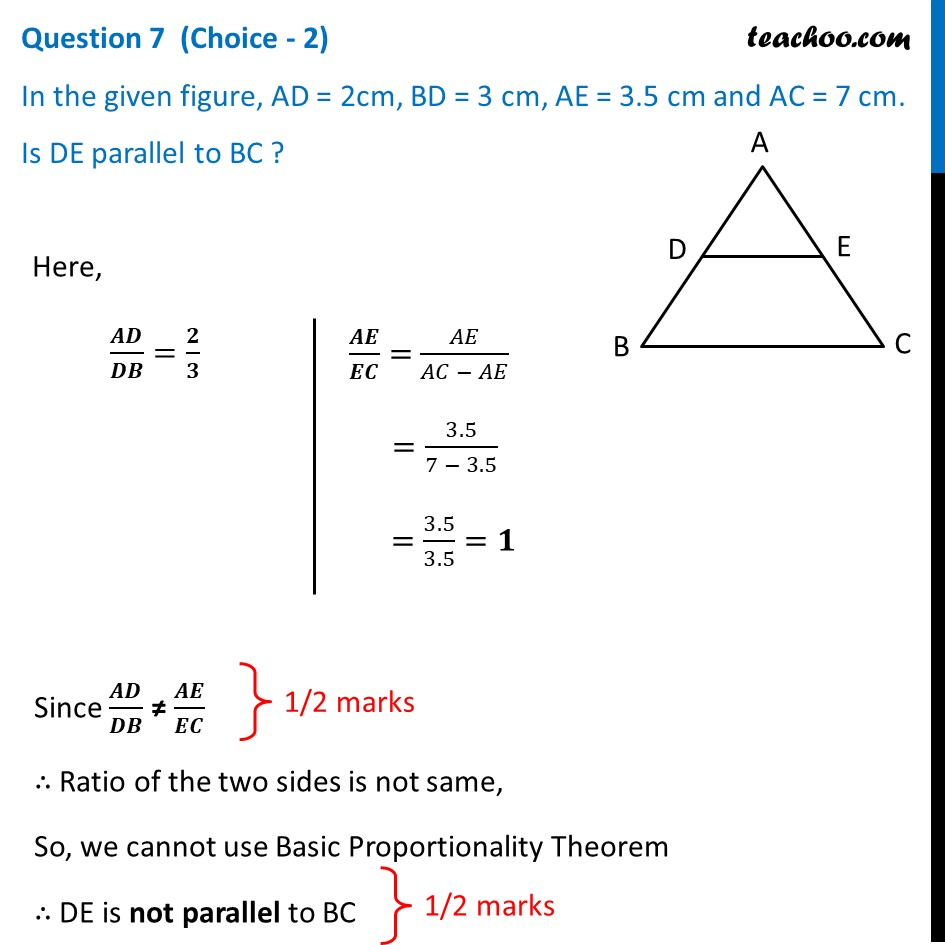## In the given figure, AD = 2cm, BD = 3 cm, AE = 3.5 cm and AC = 7 cm. Is DE parallel to BC ?1. Class 10
2. Solutions of Sample Papers for Class 10 Boards
3. CBSE Class 10 Sample Paper for 2021 Boards - Maths Basic

Transcript

Question 7 (Choice - 2) In the given figure, AD = 2cm, BD = 3 cm, AE = 3.5 cm and AC = 7 cm. Is DE parallel to BC ? Here, 𝑨𝑫/𝑫𝑩=𝟐/𝟑 𝑨𝑬/𝑬𝑪= 𝐴𝐸/(𝐴𝐶 − 𝐴𝐸) =3.5/(7 − 3.5) =3.5/3.5=𝟏 Since 𝑨𝑫/𝑫𝑩 ≠ 𝑨𝑬/𝑬𝑪 ∴ Ratio of the two sides is not same, So, we cannot use Basic Proportionality Theorem ∴ DE is not parallel to BC

CBSE Class 10 Sample Paper for 2021 Boards - Maths Basic

Class 10
Solutions of Sample Papers for Class 10 Boards